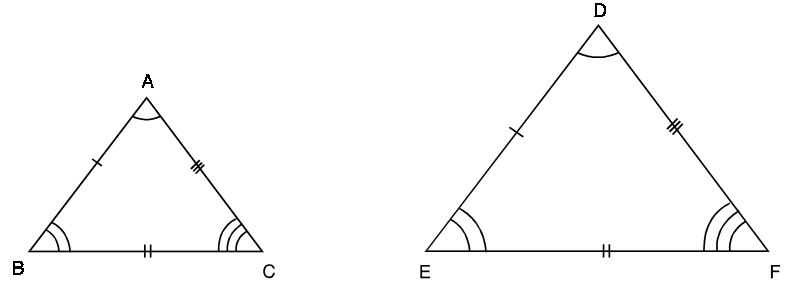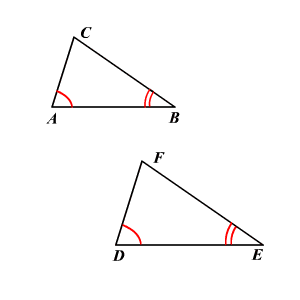The Learning Point‎ > ‎Mathematics‎ > ‎

### Similarity of Triangles

Theorems:
2.1Two figures are similar if one is the image of the other under a transformation from the plane into itself that multiplies all distances by the same positive scale factor.

2.2Definition:Two triangles are similar if the following this are hold:
a)Their corresponding angles are equal.
b)Their corresponding sides are equal.

We say that  ∆ABC is similar to ∆DEF and denote it by writing
∆ABC ~ ∆DEF.

The symbol ‘~’ stands for the phrase “ is similar to”
If ∆ABC ~ ∆DEF, then by definition
∠A = ∠D, ∠B = ∠E, ∠D = ∠F and AB/DE = BC/EF = CA/FD2.3 It can be said that all congruent triangles are similar, but all similar triangles are not congruent. The only difference is that triangles are proportional by a scalar constant k, whereas in congruence that scalar is of unit value.

2.4 Criteria for similarity:
1.AAA(Angle-Angle-Angle):If in two triangles, the corresponding angles are equal then the triangles are similar.In can be easily seen in the figure below.2.SSS(Side-Side-Side):If the corresponding sides of two triangles are proportional then the triangles are similar. It is shown that the ratio of the corresponding sides are in the ratio of ½.

3. SAS(Side-Angle-Side):If one angle of a triangle is equal to one angle of the other triangle and the sides containing these angles are proportional, then the triangles are similar.

2.7 Basic Proportionality theorem:
a)If a line is drawn parallel to one side of a triangle, the other two sides of the triangle are divided proportionally.
b)If a line divides any two sides of a triangle in the same ratio, the line is parallel to third side of the triangle.

2.6 Area of similar Triangle theorem: In two similar triangles, the ratio of their areas is the square of the ratio of their sides. In the figure shown below all the blue triangles are are similar to the larger triangle and it can be seen the above theorem holds.

2.7 Problems:

Q1.In figure below, ∆ABC ~ ∆PQR. If AC = 4.8 cm, AB = 4 cm and PQ = 9 cm, find PR.

Q2.In Figure below, ABC is a right triangle with  ∠A = 90° and  ∠C = 30°. Show that ∆DAB ~ ∆DCA ~ ∆ACB.

Q3.In two similar triangles ABC and PQR, if the corresponding altitudes AD and PS are in the ratio of 4 : 9, find the ratio of the areas of ∆ABC and  ∆PQR.

Q4.In Figure below, ABC is a triangle in which DE || BC. If AB = 6 cm and AD = 2 cm, find the ratio of the area of ∆ADE and trapezium DBCE.

Q5.If the ratio of the areas of two similar triangles is 16 : 25, find the ratio of their corresponding sides.

Q6.The sides AB and AC of a triangle are 6 cm and 8 cm. The bisector AD of ∠A intersects the opposite side BC in D such that BD = 4.5 cm. Find the length of segment CD.